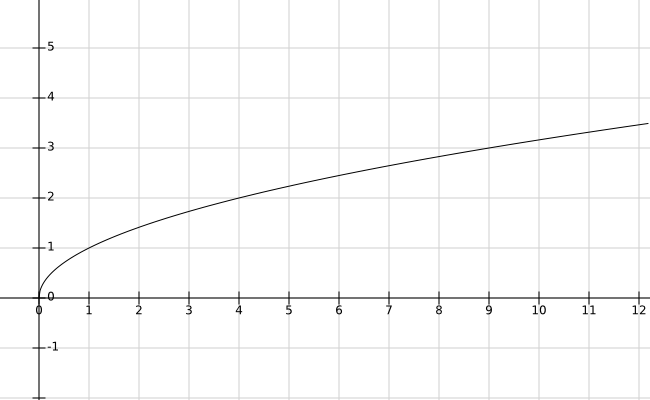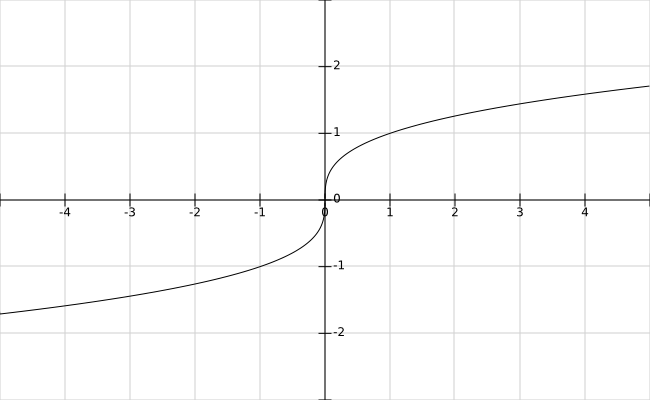# Sections 2.6 - Graphs of Special Functions; Translations

Section Objectives

1. Sketch the graph of $f(x)=ax^3$.
2. Use basic translations to sketch graphs.
3. Sketch the graphs of square root and cube root functions.
4. Sketch the graphs of shifted parabolas.

### Graphing basic cubic functions

Like lines and parabolas, the graphs of functions of the form $f(x)=ax^3$ are part of our collection of basic college algebra graphs. Before plotting any points, we can actually notice a few very important features of the graph:

• The graph of $f(x)=ax^3$ passes through the origin, $(0,0)$. It has no other $x$- or $y$-intercepts.
• $f(x)=ax^3$ is an odd function. The graph is symmetric about the origin.
• The graph is relatively flat near the origin and very steep away from the origin.
• If $a>0$, $f$ is an increasing function. If $a<0$, $f$ is a decreasing function.

With these ideas in mind, it takes only a few plotted points to obtain a very nice graph.

#### Examples

• Sketch the graph of $y=x^3$.

• Sketch the graph of $\displaystyle f(x)=-\frac{5}{4} x^3$.

### The graphs of the basic square root and cube root functions

Just as we analyzed the graph of $y=ax^3$ before we even started sketching it, we could similarly think about the graphs of $y=\sqrt{x}$ and $y=\sqrt{x}$.

Here is the graph of $f(x)=\sqrt{x}$.And here is the graph of $f(x)=\sqrt{x}$.### Translations of graphs

The following ideas will help us to easily obtain the graphs of more complicated functions from our basic graphs.

Suppose that we have the graph of the function $y=f(x)$ and that $k$ is a positive number.

• The graph of $y=f(x)+k$ is the graph of $y=f(x)$ vertically shifted $k$ units upward.
• The graph of $y=f(x)-k$ is the graph of $y=f(x)$ vertically shifted $k$ units downward.
• The graph of $y=f(x-k)$ is the graph of $y=f(x)$ horizontally shifted $k$ units right.
• The graph of $y=f(x+k)$ is the graph of $y=f(x)$ horizontally shifted $k$ units left.

#### Examples

• First describe the graph of $h(x) = \sqrt{x} + 3$. Then sketch it.

• What equation has the graph of $y=x^2$ shifted $8$ units left?

• Start with the graph of $y=\sqrt{x}$. Shift it $4$ units down, then $7$ units right. What is an equation for the new graph?

• Sketch the graph of $f(x) = (x-1)^2+2$.

• Sketch the graph of $g(x)=-2(x+2)^3+3$.